A Hierarchical model for Rugby prediction¶

:::{post} 30 Aug, 2021 :tags: hierarchical, pymc3.Data, pymc3.Deterministic, pymc3.HalfNormal, pymc3.Model, pymc3.Normal, pymc3.Poisson, sports :category: intermediate :::

Based on the following blog post: Daniel Weitzenfeld's, which based on the work of Baio and Blangiardo.

In this example, we're going to reproduce the first model described in the paper using PyMC3.

Since I am a rugby fan I decide to apply the results of the paper to the Six Nations Championship, which is a competition between Italy, Ireland, Scotland, England, France and Wales.

Motivation¶

Your estimate of the strength of a team depends on your estimates of the other strengths

Ireland are a stronger team than Italy for example - but by how much?

Source for Results 2014 are Wikipedia. I've added the subsequent years, 2015, 2016, 2017. Manually pulled from Wikipedia.

• We want to infer a latent parameter - that is the 'strength' of a team based only on their scoring intensity, and all we have are their scores and results, we can't accurately measure the 'strength' of a team.
• Probabilistic Programming is a brilliant paradigm for modeling these latent parameters
• Aim is to build a model for the upcoming Six Nations in 2018.
In :
!date

import arviz as az
import matplotlib.pyplot as plt
import numpy as np
import pandas as pd
import pymc3 as pm
import seaborn as sns
import theano.tensor as tt
import xarray as xr

from matplotlib.ticker import StrMethodFormatter

%matplotlib inline
Sat Apr  3 14:39:36 IST 2021
In :
az.style.use("arviz-darkgrid")
plt.rcParams["figure.constrained_layout.use"] = False

This is a Rugby prediction exercise. So we'll input some data. We've taken this from Wikipedia and BBC sports.

In :
try:
except:

What do we want to infer?¶

• We want to infer the latent paremeters (every team's strength) that are generating the data we observe (the scorelines).
• Moreover, we know that the scorelines are a noisy measurement of team strength, so ideally, we want a model that makes it easy to quantify our uncertainty about the underlying strengths.
• Often we don't know what the Bayesian Model is explicitly, so we have to 'estimate' the Bayesian Model'
• If we can't solve something, approximate it.
• Markov-Chain Monte Carlo (MCMC) instead draws samples from the posterior.
• Fortunately, this algorithm can be applied to almost any model.

What do we want?¶

• We want to quantify our uncertainty
• We want to also use this to generate a model
• We want the answers as distributions not point estimates

Visualization/EDA¶

We should do some some exploratory data analysis of this dataset.

The plots should be fairly self-explantory, we'll look at things like difference between teams in terms of their scores.

In :
df_all.describe()
Out:
home_score away_score year
count 60.000000 60.000000 60.000000
mean 23.500000 19.983333 2015.500000
std 14.019962 12.911028 1.127469
min 0.000000 0.000000 2014.000000
25% 16.000000 10.000000 2014.750000
50% 20.500000 18.000000 2015.500000
75% 27.250000 23.250000 2016.250000
max 67.000000 63.000000 2017.000000
In :
# Let's look at the tail end of this dataframe
df_all.tail()
Out:
home_team away_team home_score away_score year
55 Italy France 18 40 2017
56 England Scotland 61 21 2017
57 Scotland Italy 29 0 2017
58 France Wales 20 18 2017
59 Ireland England 13 9 2017

There are a few things here that we don't need. We don't need the year for our model. But that is something that could improve a future model.

Firstly let us look at differences in scores by year.

In :
df_all["difference"] = np.abs(df_all["home_score"] - df_all["away_score"])
In :
(
df_all.groupby("year")["difference"]
.mean()
.plot(
kind="bar",
title="Average magnitude of scores difference Six Nations",
yerr=df_all.groupby("year")["difference"].std(),
)
.set_ylabel("Average (abs) point difference")
);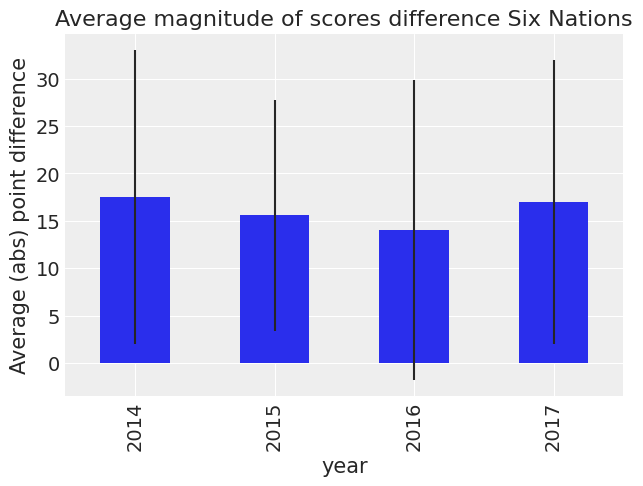We can see that the standard error is large. So we can't say anything about the differences. Let's look country by country.

In :
df_all["difference_non_abs"] = df_all["home_score"] - df_all["away_score"]

Let us first loook at a Pivot table with a sum of this, broken down by year.

In :
df_all.pivot_table("difference_non_abs", "home_team", "year")
Out:
year 2014 2015 2016 2017
home_team
England 7.000000 20.666667 7.500000 21.333333
France 6.666667 0.000000 -2.333333 4.000000
Ireland 28.000000 8.500000 17.666667 7.000000
Italy -21.000000 -31.000000 -23.500000 -33.666667
Scotland -11.000000 -12.000000 2.500000 16.666667
Wales 25.666667 1.000000 22.000000 4.000000

Now let's first plot this by home team without year.

In :
(
df_all.pivot_table("difference_non_abs", "home_team")
.rename_axis("Home_Team")
.plot(kind="bar", rot=0, legend=False)
.set_ylabel("Score difference Home team and away team")
);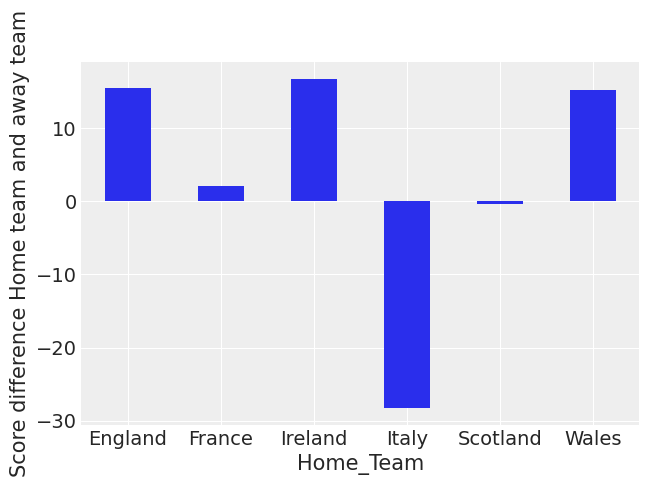You can see that Italy and Scotland have negative scores on average. You can also see that England, Ireland and Wales have been the strongest teams lately at home.

In :
(
df_all.pivot_table("difference_non_abs", "away_team")
.rename_axis("Away_Team")
.plot(kind="bar", rot=0, legend=False)
.set_ylabel("Score difference Home team and away team")
);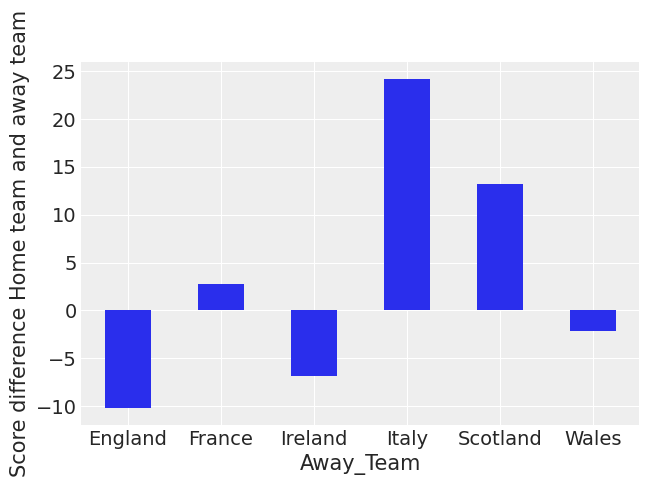This indicates that Italy, Scotland and France all have poor away from home form. England suffers the least when playing away from home. This aggregate view doesn't take into account the strength of the teams.

Let us look a bit more at a timeseries plot of the average of the score difference over the year.

We see some changes in team behaviour, and we also see that Italy is a poor team.

In :
g = sns.FacetGrid(df_all, col="home_team", col_wrap=2, height=5)
g.map(sns.scatterplot, "year", "difference_non_abs")
g.fig.autofmt_xdate()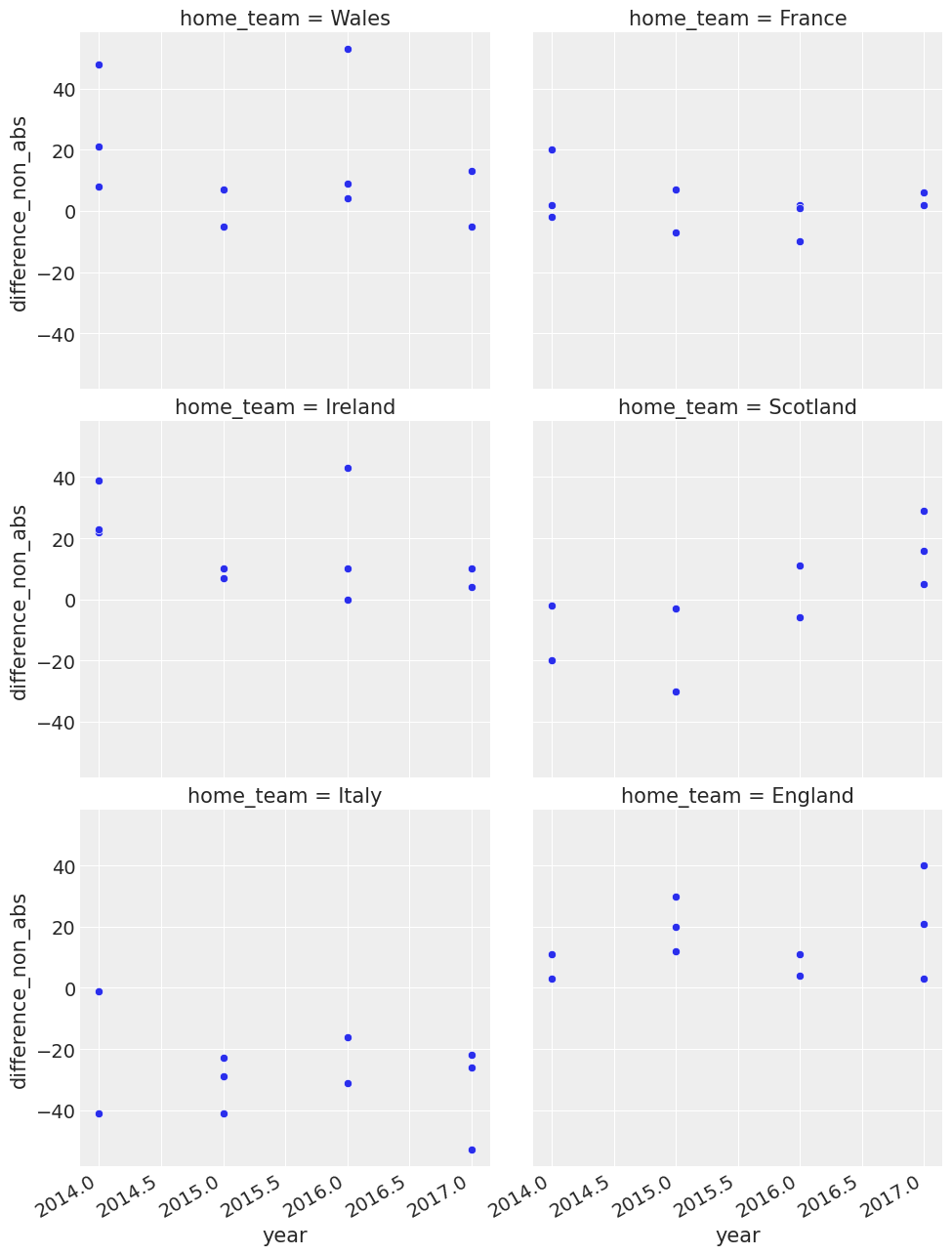In :
g = sns.FacetGrid(df_all, col="away_team", col_wrap=2, height=5)
g = g.map(plt.scatter, "year", "difference_non_abs").set_axis_labels("Year", "Score Difference")
g.fig.autofmt_xdate()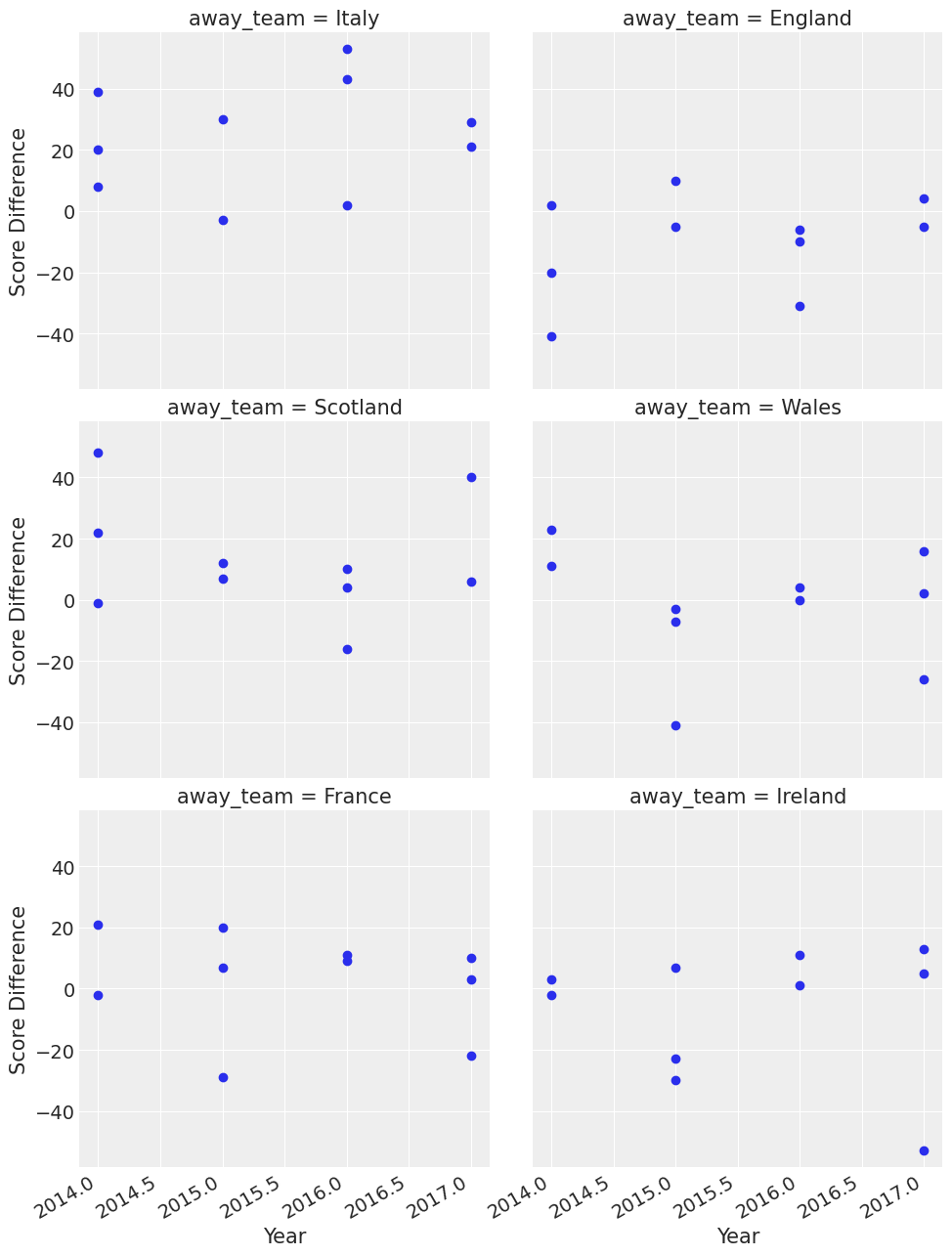You can see some interesting things here like Wales were good away from home in 2015. In that year they won three games away from home and won by 40 points or so away from home to Italy.

So now we've got a feel for the data, we can proceed on with describing the model.

What assumptions do we know for our 'generative story'?¶

• We know that the Six Nations in Rugby only has 6 teams - they each play each other once
• We have data from the last few years
• We also know that in sports scoring is modelled as a Poisson distribution
• We consider home advantage to be a strong effect in sports

The model.¶

The league is made up by a total of T= 6 teams, playing each other once in a season. We indicate the number of points scored by the home and the away team in the g-th game of the season (15 games) as $y_{g1}$ and $y_{g2}$ respectively. </p> The vector of observed counts $\mathbb{y} = (y_{g1}, y_{g2})$ is modelled as independent Poisson: $y_{gi}| \theta_{gj} \tilde\;\; Poisson(\theta_{gj})$ where the theta parameters represent the scoring intensity in the g-th game for the team playing at home (j=1) and away (j=2), respectively.</p>

We model these parameters according to a formulation that has been used widely in the statistical literature, assuming a log-linear random effect model: $$log \theta_{g1} = home + att_{h(g)} + def_{a(g)}$$ $$log \theta_{g2} = att_{a(g)} + def_{h(g)}$$

• The parameter home represents the advantage for the team hosting the game and we assume that this effect is constant for all the teams and throughout the season
• The scoring intensity is determined jointly by the attack and defense ability of the two teams involved, represented by the parameters att and def, respectively

• Conversely, for each t = 1, ..., T, the team-specific effects are modelled as exchangeable from a common distribution:

• $att_{t} \; \tilde\;\; Normal(\mu_{att},\tau_{att})$ and $def_{t} \; \tilde\;\;Normal(\mu_{def},\tau_{def})$

• We did some munging above and adjustments of the data to make it tidier for our model.
• The log function to away scores and home scores is a standard trick in the sports analytics literature

Building of the model¶

• We now build the model in PyMC3, specifying the global parameters, and the team-specific parameters and the likelihood function
In :
home_idx, teams = pd.factorize(df_all["home_team"], sort=True)
away_idx, _ = pd.factorize(df_all["away_team"], sort=True)
coords = {"team": teams, "match": np.arange(60)}
In :
with pm.Model(coords=coords) as model:
# constant data
home_team = pm.Data("home_team", home_idx, dims="match")
away_team = pm.Data("away_team", away_idx, dims="match")

# global model parameters
home = pm.Normal("home", mu=0, sigma=1)
sd_att = pm.HalfNormal("sd_att", sigma=2)
sd_def = pm.HalfNormal("sd_def", sigma=2)
intercept = pm.Normal("intercept", mu=3, sigma=1)

# team-specific model parameters
atts_star = pm.Normal("atts_star", mu=0, sigma=sd_att, dims="team")
defs_star = pm.Normal("defs_star", mu=0, sigma=sd_def, dims="team")

atts = pm.Deterministic("atts", atts_star - tt.mean(atts_star), dims="team")
defs = pm.Deterministic("defs", defs_star - tt.mean(defs_star), dims="team")
home_theta = tt.exp(intercept + home + atts[home_idx] + defs[away_idx])
away_theta = tt.exp(intercept + atts[away_idx] + defs[home_idx])

# likelihood of observed data
home_points = pm.Poisson(
"home_points",
mu=home_theta,
observed=df_all["home_score"],
dims=("match"),
)
away_points = pm.Poisson(
"away_points",
mu=away_theta,
observed=df_all["away_score"],
dims=("match"),
)
trace = pm.sample(1000, tune=1000, cores=4, return_inferencedata=True, target_accept=0.85)
Auto-assigning NUTS sampler...
Multiprocess sampling (4 chains in 4 jobs)
NUTS: [defs_star, atts_star, intercept, sd_def, sd_att, home]
100.00% [8000/8000 00:22<00:00 Sampling 4 chains, 0 divergences]
Sampling 4 chains for 1_000 tune and 1_000 draw iterations (4_000 + 4_000 draws total) took 38 seconds.
0, dim: match, 60 =? 60
0, dim: match, 60 =? 60
• We specified the model and the likelihood function

• All this runs on a Theano graph under the hood

• return_inferencedata=True stores Arviz.InferenceData in trace variable

• Note: To know more about Arviz-Pymc3 integration, see official documentation

In :
az.plot_trace(trace, var_names=["intercept", "home", "sd_att", "sd_def"], compact=False);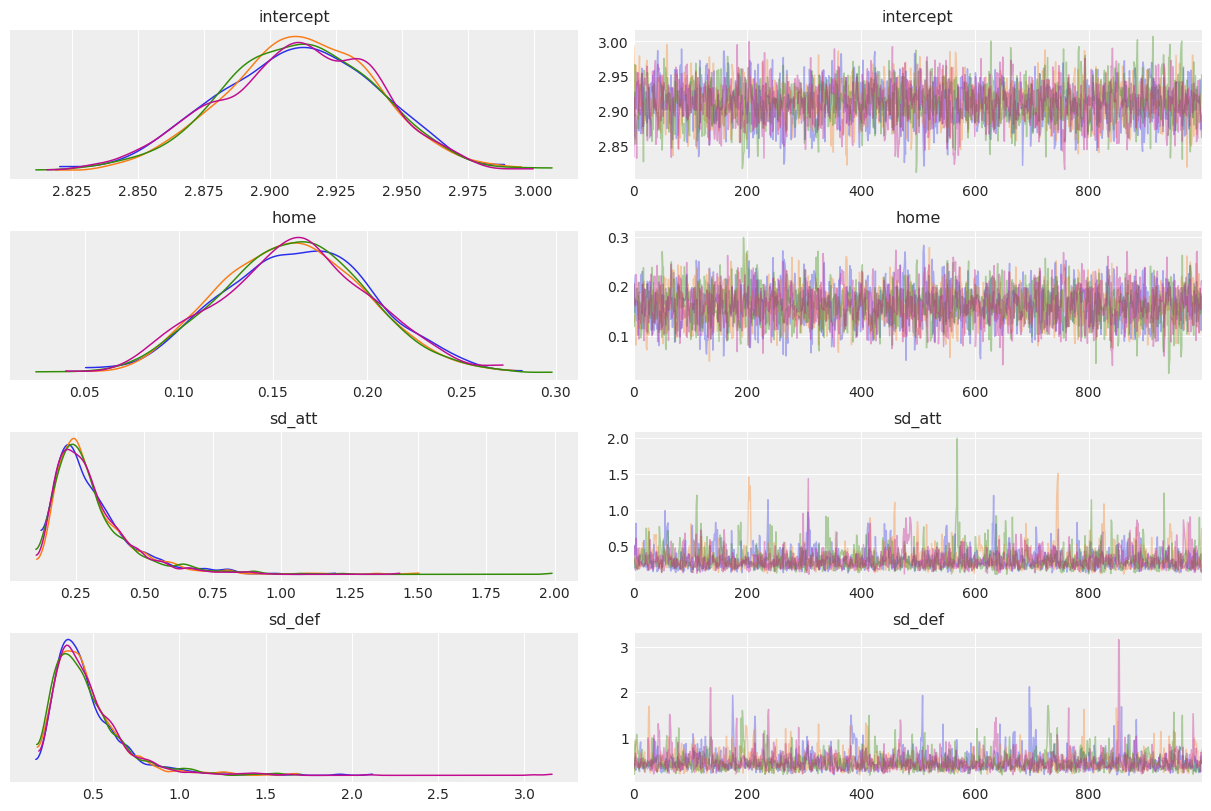Let us apply good statistical workflow practices and look at the various evaluation metrics to see if our NUTS sampler converged.

In :
az.plot_energy(trace, figsize=(6, 4));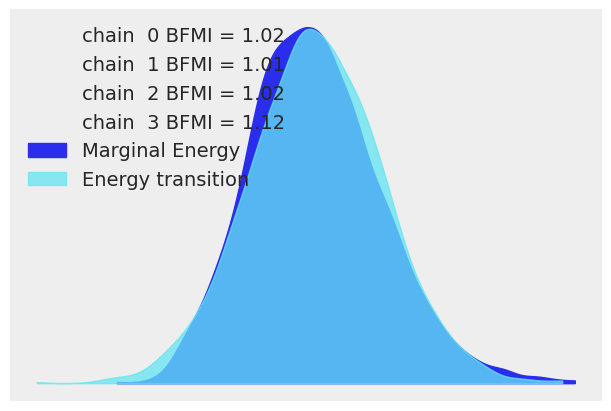In :
az.summary(trace, kind="diagnostics")
Out:
mcse_mean mcse_sd ess_bulk ess_tail r_hat
home 0.001 0.001 2807.0 2444.0 1.0
intercept 0.001 0.000 2581.0 2185.0 1.0
atts_star 0.005 0.004 1097.0 966.0 1.0
atts_star 0.005 0.004 1112.0 1024.0 1.0
atts_star 0.005 0.004 1123.0 966.0 1.0
atts_star 0.005 0.004 1144.0 1040.0 1.0
atts_star 0.005 0.004 1126.0 1010.0 1.0
atts_star 0.005 0.004 1126.0 1043.0 1.0
defs_star 0.007 0.005 1038.0 1202.0 1.0
defs_star 0.007 0.005 1145.0 1306.0 1.0
defs_star 0.007 0.005 1209.0 1299.0 1.0
defs_star 0.007 0.005 1152.0 1312.0 1.0
defs_star 0.007 0.005 1188.0 1324.0 1.0
defs_star 0.007 0.005 1159.0 1285.0 1.0
sd_att 0.004 0.003 1942.0 1746.0 1.0
sd_def 0.005 0.004 2047.0 1810.0 1.0
atts 0.001 0.000 4390.0 2771.0 1.0
atts 0.001 0.000 4843.0 2409.0 1.0
atts 0.001 0.000 4764.0 3215.0 1.0
atts 0.001 0.001 4834.0 3208.0 1.0
atts 0.001 0.001 4735.0 2857.0 1.0
atts 0.001 0.000 5146.0 3101.0 1.0
defs 0.001 0.001 4074.0 3326.0 1.0
defs 0.001 0.001 4650.0 3654.0 1.0
defs 0.001 0.001 4875.0 3297.0 1.0
defs 0.001 0.000 4362.0 3431.0 1.0
defs 0.001 0.000 4163.0 2936.0 1.0
defs 0.001 0.000 4839.0 3141.0 1.0

Our model has converged well and $\hat{R}$ looks good.

Let us look at some of the stats, just to verify that our model has returned the correct attributes. We can see that some teams are stronger than others. This is what we would expect with attack

In :
trace_hdi = az.hdi(trace)
trace_hdi["atts"]
Out:
<xarray.DataArray 'atts' (team: 6, hdi: 2)>
array([[ 0.18081206,  0.33212389],
[-0.1657255 ,  0.00654105],
[ 0.03330137,  0.19106398],
[-0.44408449, -0.23789269],
[-0.20479866, -0.03114821],
[ 0.1000778 ,  0.25062539]])
Coordinates:
* team     (team) object 'England' 'France' 'Ireland' ... 'Scotland' 'Wales'
* hdi      (hdi) <U6 'lower' 'higher'
In :
trace.posterior["atts"].median(("chain", "draw"))
Out:
<xarray.DataArray 'atts' (team: 6)>
array([ 0.25623782, -0.08372123,  0.10803372, -0.33362557, -0.11699874,
0.17168809])
Coordinates:
* team     (team) object 'England' 'France' 'Ireland' ... 'Scotland' 'Wales'

Results¶

From the above we can start to understand the different distributions of attacking strength and defensive strength. These are probabilistic estimates and help us better understand the uncertainty in sports analytics

In :
_, ax = plt.subplots(figsize=(12, 6))

ax.scatter(teams, trace.posterior["atts"].median(dim=("chain", "draw")), color="C0", alpha=1, s=100)
ax.vlines(
teams,
trace_hdi["atts"].sel({"hdi": "lower"}),
trace_hdi["atts"].sel({"hdi": "higher"}),
alpha=0.6,
lw=5,
color="C0",
)
ax.set_xlabel("Teams")
ax.set_ylabel("Posterior Attack Strength")
ax.set_title("HDI of Team-wise Attack Strength");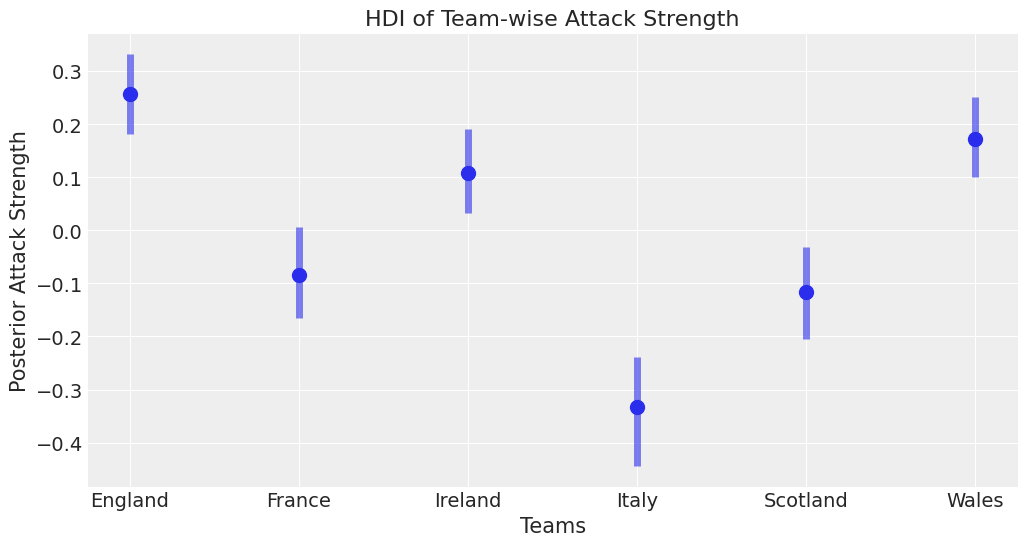This is one of the powerful things about Bayesian modelling, we can have uncertainty quantification of some of our estimates. We've got a Bayesian credible interval for the attack strength of different countries.

We can see an overlap between Ireland, Wales and England which is what you'd expect since these teams have won in recent years.

Italy is well behind everyone else - which is what we'd expect and there's an overlap between Scotland and France which seems about right.

There are probably some effects we'd like to add in here, like weighting more recent results more strongly. However that'd be a much more complicated model.

In :
ax = az.plot_forest(trace, var_names=["atts"])
ax.set_yticklabels(teams)
ax.set_title("Team Offense");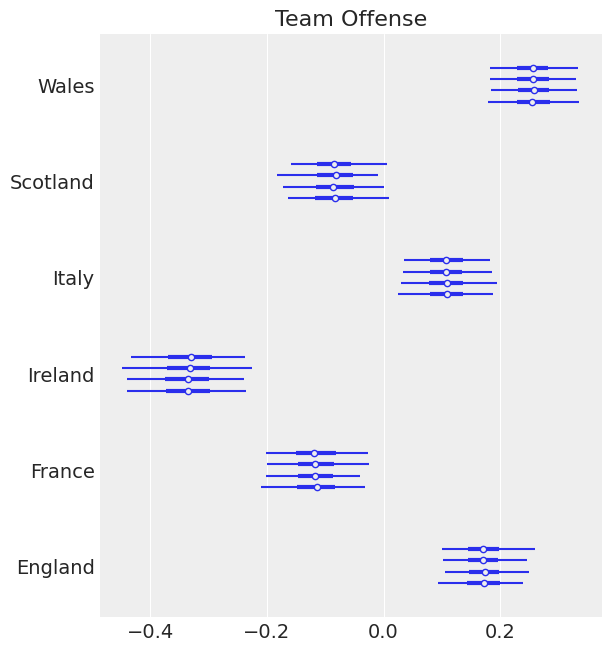In :
ax = az.plot_forest(trace, var_names=["defs"])
ax.set_yticklabels(teams)
ax.set_title("Team Defense");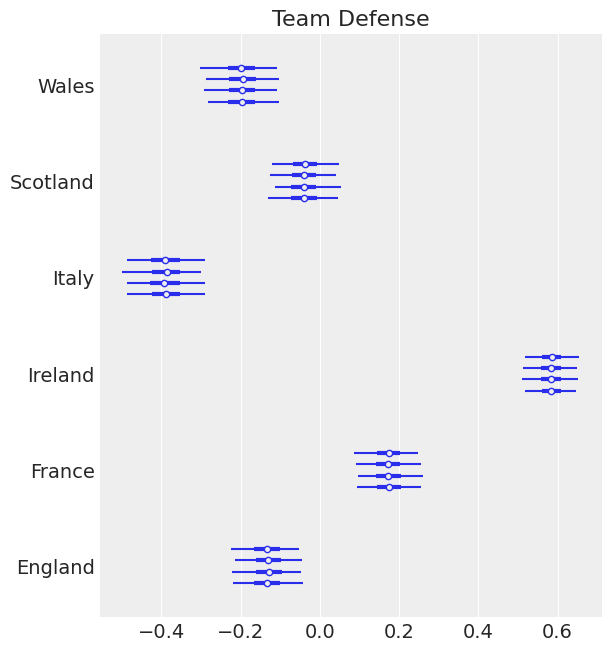Good teams like Ireland and England have a strong negative effect defense. Which is what we expect. We expect our strong teams to have strong positive effects in attack and strong negative effects in defense.

This approach that we're using of looking at parameters and examining them is part of a good statistical workflow. We also think that perhaps our priors could be better specified. However this is beyond the scope of this article. We recommend for a good discussion of 'statistical workflow' you visit Robust Statistical Workflow with RStan

Let's do some other plots. So we can see our range for our defensive effect. I'll print the teams below too just for reference

In :
az.plot_posterior(trace, var_names=["defs"]);